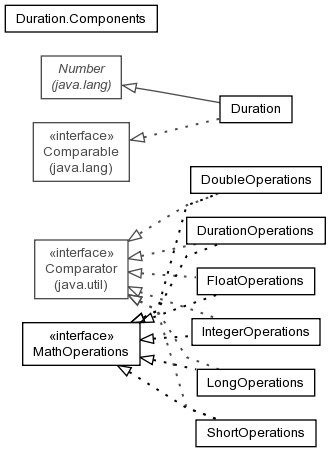## Package org.modeshape.common.math

A set of classes that define mathematic operations for a particular class of values.

See:
DescriptionInterface Summary
MathOperations<T> The set of mathematic operations for a particular class of values.

Class Summary
DoubleOperations The `math operations` for double numbers.
Duration A number representing an immutable duration of time.
DurationOperations The `math operations` for `Duration`s.
FloatOperations The `math operations` for float numbers.
IntegerOperations The `math operations` for integer numbers.
LongOperations The `math operations` for long numbers.
ShortOperations The `math operations` for short numbers.

## Package org.modeshape.common.math Description

A set of classes that define mathematic operations for a particular class of values. This is useful for generic classes that must work with one of the `Number` subclasses.

Copyright © 2008-2010 JBoss, a division of Red Hat. All Rights Reserved.